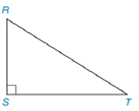Chapter 7.1, Problem 10E### Elementary Geometry for College St...

6th Edition
Daniel C. Alexander + 1 other
ISBN: 9781285195698

#### Solutions

Chapter
Section### Elementary Geometry for College St...

6th Edition
Daniel C. Alexander + 1 other
ISBN: 9781285195698
Textbook Problem
1 views

# Note: Exercises preceded by an asterisk are of a more challenging nature.In Exercises 3 to 8, use the drawing provided.Given: Right ∆ R S T Construct: The median R to S T -

To determine

To construct:

The median R to ST-

Explanation

Definition:

Perpendicular bisector of a triangle is a line passing through the midpoint of the particular side of the triangle.

Calculation:

The obtuse ABC is given

To construct a bisector we follow the below procedure.

Step 1: Construct a right angle triangle RST.

Step 2: Locate the midpoint of the side ST and label it as D.

Step 3: Draw a line from the point R to the point D

### Still sussing out bartleby?

Check out a sample textbook solution.

See a sample solution

#### The Solution to Your Study Problems

Bartleby provides explanations to thousands of textbook problems written by our experts, many with advanced degrees!

Get Started

#### In Exercises 19-24, find the functions f + g, f g, fg, and fg. 19. f(x) = x2 + 5; g(x) = x2

Applied Calculus for the Managerial, Life, and Social Sciences: A Brief Approach

#### Determine the infinite limit. limx12x(x1)2

Single Variable Calculus: Early Transcendentals

#### True or False:

Study Guide for Stewart's Multivariable Calculus, 8th